# Ratio Worksheets

#### What are Ratios?

Ratios are clarified mainly are based upon fractions. Whenever a fraction is signified in the form of x:y, then it turns into a ratio; however, a proportion is when two ratios are alike. Here x and y are any two integers. Proportions and ratios go side by side. Ratios are the foundation through which you can understand many mathematics concepts and some science concepts. We are using the concept of ratios in our lives daily, such as in a business when we are dealing with money, not only in business but also in our house cooking in the kitchen.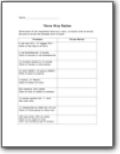###### Three Way Ratios

Write each of the requested ratios as a ratio, a fraction and as words. Be sure to write the simplest form of each.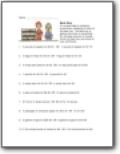It's a good idea to compare prices when shopping to look for the best buy. The best buy is getting the most of something for the least amount of money. Circle the best buy and show all your arithmetic.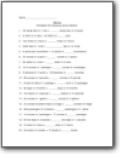###### Fill in to Complete

Complete the following ratio problems.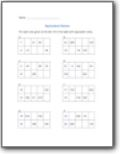###### Equivalent Ratios

For each ratio given at the left, fill in the table with equivalent ratios.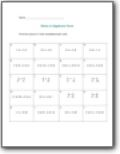###### Ratio in Algebraic Form

Find the value of n that completes each ratio.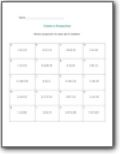###### Create a Proportion

Write a proportion for each set of numbers.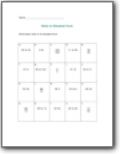###### Ratio in Simplest Form

Write each ratio in its simplest form.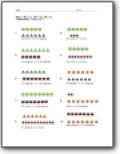###### Writing Ratios

Write the ratio of the relationship between the number of shapes that are present.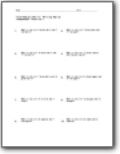###### Ratio Word Problems

Breakdown the word problems to help make sense of them.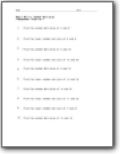###### Common Multiples

You will find the common multiples between two numbers.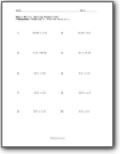###### Solving Proportions

Find the value of x.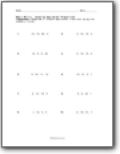###### Creating Equivalent Proportions

Create equivalent fractions using the numbers listed.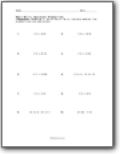###### Equivalent Propositions

Write Yes or No to indicate whether the propositions are equivalent.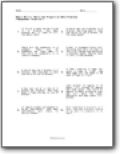###### Ratio and Proportion Word Problems

You will solve problems such as: Jack picked 12 apples & 15 pears. Jill picked 16 apples and some pears. The ratio of apples to pears picked by Jack and Jill were the same. Determine how many pears Jill picked.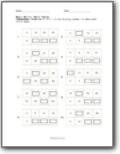###### Ratio Tables

Fill in the missing number to make each ratio equal.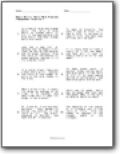###### Ratio Intermediate Word Problems

We will solve a word problems such as: When a birds flies, it beats its wings an average of 40 times in 30 seconds. How many times will it beat its wings in two minutes?